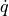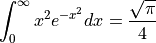# @f{latex}¶

Displays a LaTeX Formula in a Text object, axis title of table cell.

@f{latex(LatexFormula)}
@f{latex(LatexFormula, DisplayParameter)}
@f{latex(LatexAliasName)}
@f{latex(LatexAliasName, DisplayParameter)}
LatexFormula

The formula can be entered directly. However, the formula must be be written in one line and begin with double $characters and end with double$ characters (display math mode). Example:

@f{latex($$\dot{m}$$)}


Instead of entering the formula directly, you can also enter the formula in a file, see Tools=>LaTeX Formula Configuration. The formula can then be multiline, and is selected by its name.

LatexAliasName

Is the name of an LaTeX-Fomula, defined in Tools=>LaTeX Formula Configuration. Example:

@f{latex(m_dot)}

DisplayParameter

The LaTeX formula is displayed as an PNG image. The size of the image can be specified with the following parameters. The default parameter is height = 1em. The em unit is the font size of the selected font. You can also enter 1.5em.

Display Parameters:

Parameter Abbreviation Description
name n Image name as displayed in the attachment dialog box.
width w Image width.
height h Image height.
xoffset x Horizontal offset
yoffset y Vertical offset
keepratio k If set to k=1, the image aspect ratio is kept. For k=0 the height and width can be set separately. k=1 is the default value.

Units for height, width and offset:

Unit Description
em Unit is the font height. The font height depends on the selected font.
cm Unit is centimeter.
mm Unit is millimeter.
in Unit is inch.
pc Unit is pica (1/6 inch).
pt Unit is point (1/72 inch).
% Unit is percent of original size (0 to 1000%).

Comment

To create new LaTeX formulas, LaTeX must be installed on your computer. You can find information about the installation under ref:edit-insert-formula.

For each formula, a PNG image file is created using the command Tools=>LaTeX Formula Configuration. The name of the formula is passed to the field function as the first parameter.

Example

Use the command Tools=>LaTeX Formula Configuration to add the following line to the latext configuration:

q_dot = \dot{q}


To display the formula, type the following into a text object:

@f{latex(q_dot, h=1em)}


Output:Example

Use the command Tools=>LaTeX Formula Configuration to add the following line to the latext configuration:

.. code-block:: none

equation1 = $$int_{0}^{infty} x^2 e^{-x^2} dx = frac{sqrt{pi}}{4}$$

To display the formula, type the following into a text object:

@f{latex(equation1, h=2em)}


Output:Example

The LateX expression can also be passed directly as a first parameter:

@f{latex($$\dot{m}$$, h=1.5em)}


id-1870472